# ISEE Middle Level Math : Median

## Example Questions

### Example Question #41 : Median

Find the median for this set of numbers: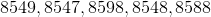None of these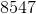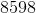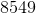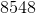Explanation:

First, order the numbers from least to greatest: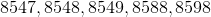Then, identify the middle number. Since there are five numbers, the middle number will be the third from either end:Answer: The median is### Example Question #42 : How To Find Median

Find the median for this set of numbers: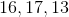None of theseExplanation:

First order the numbers from least to greatest: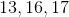Then, identify the middle number:Answer: The median is.

### Example Question #43 : How To Find Median

Find the median in this set of numbers: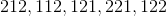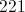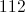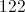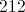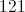Explanation:

First, order the numbers from least to greatest: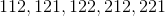Then, identify the middle number: 122

### Example Question #44 : How To Find Median

Find the median for this set of numbers: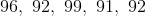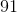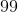None of these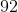Explanation:

First, order the numbers from least to greatest: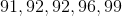The median is the number closest to the middle of the ordered set. Since there are five numbers, the median will be the third number from either side.

Answer: The median is:.

### Example Question #45 : How To Find Median

Find the median in this set of numbers: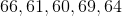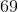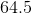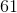Explanation:

First order the numbers from least to greatest: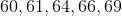Then, identify the middle number:Answer: The median is.

### Example Question #41 : Median

Find the median in this set of numbers: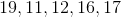Explanation:

First order the numbers from least to greatest: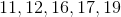Identify the middle number:.

Answer: The median is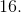### Example Question #47 : How To Find Median

Find the median in this set: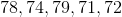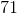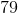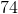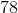Explanation:

First, order the numbers from least to greatest: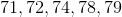Then, identify the middle number:Answer: The median is.

### Example Question #42 : Median

Find the median for this set of numbers: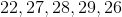Explanation:

First, order the numbers from least to greatest: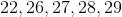Then, identify the middle number. Since there are five numbers in the set, the median will be the third number from either side:.

Answer:.

### Example Question #49 : How To Find Median

Find the median in this set: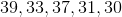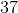Explanation:

First order the number from least to greatest: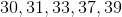Identify the middle number:Answer: The median is.

### Example Question #50 : How To Find Median

Find the median for this set of numbers: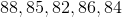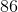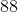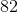Explanation:

First, order the numbers from least to greatest: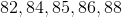Then, identify the middle number. Since there are five numbers in the set, the middle number will be the third from either end:Answer:### All ISEE Middle Level Math Resources